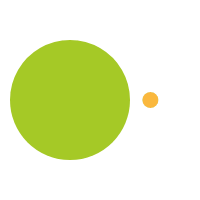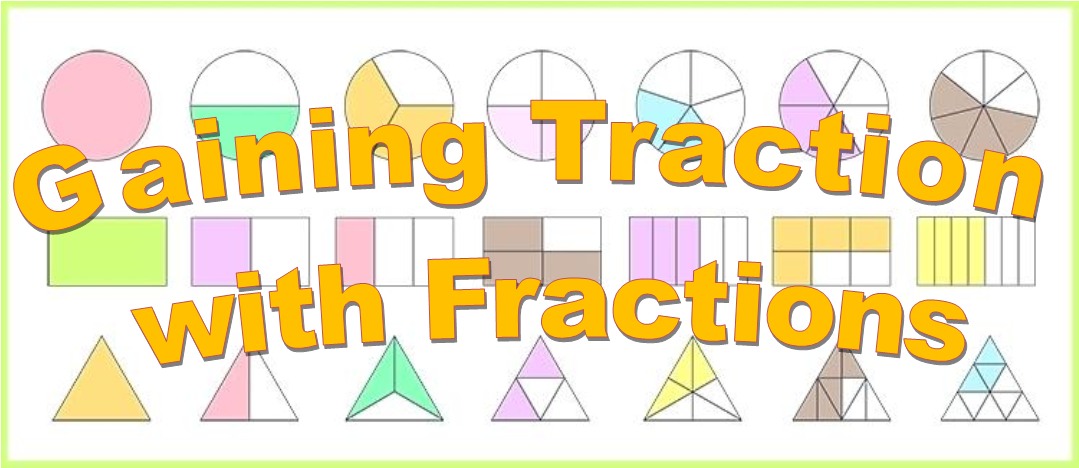# Fraction Traction - Gaining understanding in 3rd through 8th grade

February 28, 2019

# Building an understanding of fractions

In the primary grades fractions are introduced as a part of a whole with identifying simple 1/4 , 1/2 , 1/3 .  Student get a basic understanding of equivalent fractions if they can play around with manipulative like pattern blocks, fraction blocks, etc.

Now we take a step further and develop an understanding of adding, subtracting, multiply and dividing fractions and make it so students understand what they are doing while having fun.

## Understanding factors and multiples.

Students need to be fluid with the multiplies and factors up to 100.  They need to see 24 and think to themselves that 2, 3, 4, 5, 6, 12 are all factors of that number.  See a 3 and know that 6, 9, 12, 15, 18, 21, 24, 27, 20 are multiples of that number.  To establish this fluency there is a great game, Tic-Fact-Toe.  The bottom row of blue numbers are the multipliers students will be working with to create products.  Students will then cover the products on the game board.  The object of the game is to get 4 in a row.  Challenge is that students can move only one of the two counters on the multipliers row.  The game is a great way to get students to become fluid with their factors and multiples.

Developing this fluidity gives students a step up when they start finding common denominators or are simplifying fractions.

Another concept students can develop to help with fraction fluidity is divisibility.  Let’s look at some common characteristics of numbers;

Students can explore these concepts and discover divisibility which is amazing to see the relationships of numbers.  Using a multiplication board helps with identifying the patterns.

## Unit Fractions:

Unit fractions are addressed in 3rd grade and we addressed them in our blog For the Love of Fractions. A unit fraction is a fraction with a numerator of 1. This allow students to focus on one part of the fraction at a time.  Pattern blocks is a great way to build understanding of until fractions.  The yellow hexagon is one whole, red trapezoid is 1/2 , blue rhombus is 1/3 , brown polygon is 1/4  , and green triangle is 1/6 .  This helps emphasize that the numerator is simply a count of units and any fraction can be thought of as a multiple of its unit fraction.

Have students count by unit fractions: 1 one-sixth, 2 one-sixth, etc. to emphasize the role of the unit.  If students are working with pattern blocks, they may notice that the yellow hexagon is the same as 3 one-sixths, and 1 one-half.  Discuss that and any other discoveries.

## Whole:

Discuss the whole.  It can be anything; a glass, a pizza, a piece of paper, and a fraction describes equal parts of that whole.  There is no such thing as a “bigger half”.  Have the students fold paper and create the Gallon Man (attend to precision, parts must be equal).

Draw attention that the size of the fraction depends on the whole it’s related to, if I order a small pizza and give you half, is that the same amount as if I ordered a large pizza and gave you half?  Is possible for 1/3 to be bigger than 1/2  if the wholes are different.

Equal parts does not necessarily mean they’re all the same shape.  A half cup of milk poured into a tall thin glass will look different than if it’s poured into a short wide glass.

## Number lines:

Number lines show a distance from zero to a whole number, with each whole divided into parts which is consistent across all whole numbers.  An elementary ruler is a great tool to show this, the 4-in-1 ruler has four different measuring breakdowns; inches, inches to nearest 1/2 , and inches to nearest 1/4 .  Having students fold a paper to create number line allows the understanding of equivalent fractions.

Avoid using circles as students struggle with partitioning evenly and it doesn’t show the infinity of the real number system that is used across grade levels.

Pirates Booty is a good activity for practice understanding of the number system.

## Decimals:

Decimals are introduced in the fourth grade and need to be related to fractions as they ARE fractions.  They are a part of a unit but the unit is hidden in the place value notation.  Many students will see 0.4 and read it “zero point four”, instead of what it is which is 4 one-tenths and that 0.27 is 27 one-hundredths or 2 one-tenths and 7 one-hundredths.   We will address decimals more in a future blog post.

## Common Units – adding and subtracting:

When adding or subtracting anything. including fractions, we must find first the common unit.  This allows us to know how many parts to the whole.  This is sometimes referred to as “finding the common denominator”.

Sometimes the common unit is stated  1/4  +  3/4 = ?  we can add the numerators (number of parts) together over the number of units.

But what if our units aren’t obvious?

Example: 4 apples + 5 bananas = number of what? Apples? Bananas? Pieces of fruit?

there are 9 pieces of fruit (our common unit)

Number lines are a great way of showing common units as well as area models.

Here is an example:

Candy caper – using chocolate bars.

When creating the visual fraction make sure to have the lines for the first fraction run vertically and the second fraction run horizontally.

Adding and subtracting fractions and discovering common units/denominators is fun to do with chocolate bars.

After visual representations, review the fractions, what do the students notice between the unlike units and the like units.  How do we get the common unit number?  All that work, with developing fluency with factor and multiplies, brings eye opening awareness of numbers!

## Multiplying fractions:

Multiplying fractions is finding a part of a part.  If there is a half-price sale and the item is being further reduced by one-fourth, we are multiplying fractions.  That is why we often use the “of” word instead of the multiplying word,  “1/4 of  1/2”.

In our Frog Action game, we use counters to show how we multiply fractions with a whole number.

1/4 of 8 = ?

I have eight objects

I need to divide this object into 4 unit (they can be divided up either way).

The numerator tells the number of parts, in this example the answer would be 2.

1/4 x 8 = 2

Students can use a number line to develop their multiplication of fractions sense.

1/3  x   1/2 = ?

Students create ½ on their number line, divide the line segment into three equal sections.  Then figure out how many of those equal sections make up the whole.

## Dividing Fractions:

This answers the question of how many of one part/fraction is in another part/fraction.  Food is a good model and providing students with measuring cups and bags of rice or cereal is a fun for demonstration.   How many  1/3 cup servings are in a box holding 2 1/2  cups?  2 1/2  ÷ 3/4

2  1/2 cups

2  1/2 cups divided into 3/4 cups  = 3 groups of 3/4 cups and

What is left is this, 2 out of 6 or  2/6 or  1/3.

Answer   2 1/2  ÷ 3/4 = 3  1/3

It is important to show the visual, teachers often talk about find the reciprocal or inverse and many students, including myself, never understood anything that was happening and often forgot which number was to be inverse.  It became a mess.  The phrase “ours is not to reason why; just invert and multiply” does nothing to help students understand math.

Notice in this last problem that the dividend is larger than the divisor the answer is a larger mixed number.

Another fraction problem can be something like this:  There is 4/5 a pound of peanuts and John, Jill, Juan and Michelle want to share it evenly among themselves.  How much will each receive?

Problem is  4/5  ÷ 4 = ?

4/5  lbs                                                                                                           4/5 lb divided by 4 people

Creates what the whole pound looks like and how much each person receives, represented by the column.  Each person will receive 4 parts out of the 20 = 4/20 = 1/5 lb

Answer: 4/5  ÷ 4 = 1/5

What happens when the dividend is smaller than the divisor?  This question requires the student to think analytically.  What is happening with the fractions?  This goes a long way to helping students solve story problems and helping to expand exploration in the STEM fields.